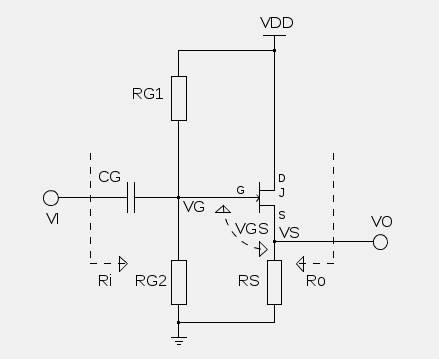OVERVIEW This is a simple design tool for calculating bias resistor values, small-signal gain and input/output resistances of a source follower JFET amplifier. The source follower is typically used as a buffer, which provides high input impedance and low output impedance. Just fill the input fields below in given order from top to bottom. The ordering of the fields serves as a step-by-step guide for the design process. The resistor values are calculated automatically using 5% tolerance resistances. The biasing will aim for a DC drain current of Idss/2 (this is set internally) and this also defines the gate-to-source voltage Vgs automatically. The small-signal values are evaluated in the mid-band, CG short-circuited. The small-signal gain is evaluated as VO/VI, and the input/output resistances are evaluated as shown in the schematic. Typical characteristic values for a n-type JFET are given as: Idss = 0.005, Vp = -1.8, for example.Select the operating voltage VDD. Typically 9 volts is the way to go. VDD : volts RESULTS DC Bias voltages: VD : volts VS : volts VG : volts Gate resistors: RG1 : ohms RG2 : ohms Source resistor: RS : ohms AC Gain factor: Av : const Input resistance: Ri : ohms Output resistance: Ro : ohms Measure the saturation current Idss of the device by connecting source and gate to ground and placing a 10 ohm resistor between drain and Vdd. Use a multimeter to measure the voltage drop over the resistor and use Ohm's law to convert the measured voltage into current. Alternatively, select a typical value of Idss from the datasheet. Idss : amps Measure the pinch-off voltage Vp of the device by connecting gate to ground, drain to Vdd and a 1 megohm resistor between source and ground. Use a multimeter to measure the voltage drop over the resistor. The voltage drop is quite close to Vp. Alternatively, select a typical value of Vp from the datasheet. Vp : volts Choose the source bias voltage to aim with the biasing design. In a normal design the source bias voltage is about VDD/2 or slightly above that. VS : volts Choose the gate resistance RG. This resistance value will be used for the small-signal analysis and for evaluating the values for resistors RG1 and RG2. RG : ohms For gain analysis, the internal output resistance of the JFET device is needed. This is usually found from the datasheet as output admittance Yos, where the output resistance is the inverse, rd = 1/Yos. rd : ohms